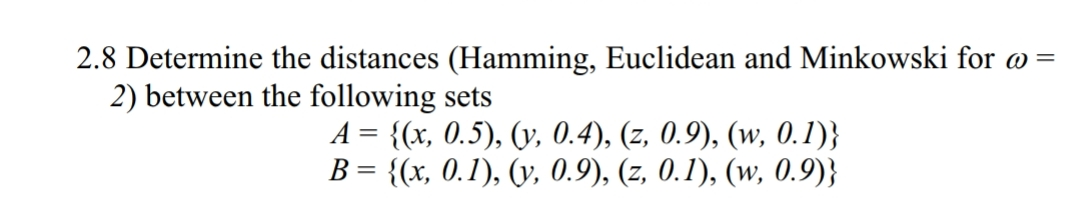# 2.8 Determine the distances (Hamming, Euclidean and Minkowski for a-2) between the following setsA-Ох, 0.5), 0, 0.4), (z, 0,9), (w, 0.1)}

Question
18 views

the book is "First Course on Fuzzy Theory and Applications"help_outlineImage Transcriptionclose2.8 Determine the distances (Hamming, Euclidean and Minkowski for a- 2) between the following sets A-Ох, 0.5), 0, 0.4), (z, 0,9), (w, 0.1)} fullscreen
check_circle

star
star
star
star
star
1 Rating
Step 1

The formula for calculating the Hamming distance (d) of fuzzy set:

Step 2

The formula for calculating the Euclidean distance (e) of fuzzy set:

Step 3

The formula for calculating the Minikowski d...

### Want to see the full answer?

See Solution

#### Want to see this answer and more?

Solutions are written by subject experts who are available 24/7. Questions are typically answered within 1 hour.*

See Solution
*Response times may vary by subject and question.
Tagged in

### Computer Science